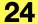Contains the Structural Analytical Model Analytical Link Rotation values.

```    typedef struct {
API_AnalyticalRigidityType     kxxType;
double                         kxxValue;
API_AnalyticalRigidityType     kyyType;
double                         kyyValue;
API_AnalyticalRigidityType     kzzType;
double                         kzzValue;
bool                           resistanceMx;
bool                           resistanceMy;
bool                           resistanceMz;
double                         mxValue;
double                         myValue;
double                         mzValue;
```

#### Members

kxxTypeRigidity type in X direction.
kxxValueRigidity value in X direction.
kyyTypeRigidity type in Y direction.
kyyValueRigidity value in Y direction.
kzzTypeRigidity type in Z direction.
kzzValueRigidity value in Z direction.
resistanceMxResistance value along the X axis.
resistanceMyResistance value along the Y axis.
resistanceMzResistance value along the Z axis.
mxValueRotation value in X direction.
myValueRotation value in Y direction.
mzValueRotation value in Z direction.

#### Requirements

Version: API 24 or later